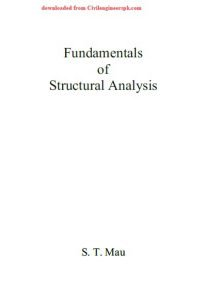# Book Fundamentals of Structural Analysis

Book Fundamentals of Structural Analysis By S. T. MauContents of book Fundamentals of Structural Analysis By S. T. Mau

Preface v
Truss Analysis: Matrix Displacement Method 1
1. What is a Truss? 1
2. A Truss Member 3
3. Member Stiffness Equation in Global Coordinates 4
Problem 1. 11
4. Unconstrained Global Stiffness Equation 11
5. Constrained Global Stiffness Equation and Its Solution 17
Problem 2. 19
6. Procedures of Truss Analysis 20
Problem 3. 27
7. Kinematic Stability 27
Problem 4. 29
8. Summary 30
Truss Analysis: Force Method, Part I 31
1. Introduction 31
2. Statically Determinate Plane Truss types 31
3. Method of Joint and Method of Section 33
Problem 1. 43
Problem 2. 55
4. Matrix Method of Joint 56
Problem 3. 61
Problem 4. 66
Truss Analysis: Force Method, Part II 67
5. Truss Deflection 67
Problem 5. 81
6. Indeterminate Truss Problems – Method of Consistent Deformations 82
7. Laws of Reciprocity 89
8. Concluding Remarks 90
Problem 6. 91
Beam and Frame Analysis: Force Method, Part I 93
1. What are Beams and Frames? 93
2. Statical Determinacy and Kinematic Stability 94
Problem 1. 101
3. Shear and Moment Diagrams 102
4. Statically Determinate Beams and Frames 109
Problem 2. 118
Beam and Frame Analysis: Force Method, Part II 121
5. Deflection of Beam and Frames 121
Problem 3. 132
Problem 4. 143
Problem 5. 152
Beam and Frame Analysis: Force Method, Part III 153
6. Statically Indeterminate Beams and Frames 153
Table: Beam Deflection Formulas 168
Problem 6. 173
Beam and Frame Analysis: Displacement Method, Part I 175
1. Introduction 175
2. Moment Distribution Method 175
Problem 1. 207
Table: Fixed End Moments 208
Beam and Frame Analysis: Displacement Method, Part II 209
3. Slope-Deflection Method 209
Problem 2. 232
4. Matrix Stiffness Analysis of Frames 233
Problem 3. 248
Influence Lines 249
1. What is Influence Line? 249
2. Beam Influence Lines 250
Problem 1. 262
3. Truss Influence Lines 263
Problem 2. 271
Other Topics 273
1. Introduction 273
2. Non-Prismatic Beam and Frames Members 273
Problem 1. 277
3. Support Movement, Temperature, and Construction Error Effects 278
Problem 2. 283
4. Secondary Stresses in Trusses 283
5. Composite Structures 285
6. Materials Non-linearity 287
7. Geometric Non-linearity 288
8. Structural Stability 290
9. Dynamic Effects 294
Matrix Algebra Review 299
1. What is a Matrix? 299
2. Matrix Operating Rules 300
3. Matrix Inversion and Solving Simultaneous Algebraic Equations 302
Problem 307
Solution to Problems 309
Index 323